Checkout JEE MAINS 2022 Question Paper Analysis : Checkout JEE MAINS 2022 Question Paper Analysis :

# Important Questions Class 10 Maths Chapter 12 Areas Related to Circles

Important questions for Class 10 Maths Chapter 12 Areas Related to Circles are given here based on the new pattern of CBSE. Students preparing for the board exams can practice these questions of Areas Related to Circles For Class 10 to score full marks for the questions from this chapter. We have also provided detailed solutions, along with the Important questions of Class 10 Maths Chapter 12 Areas Related to Circles. Students can refer to the solutions whenever they get stuck solving a problem. Also, in the end, some practice questions are provided for students to boost their preparation.

This chapter contains many formulas and concepts. Thus, it is important from the examination perspective since most of the questions, and objective-based questions will come in the exam from this chapter. Students can also refer to these important questions as a part of their exam preparation. NCERT Solutions are also available at BYJU’S to help the students in scoring full marks.

Students can also get access to Class 10 Maths Chapter 12 Areas Related Circles MCQs here.

## Important Questions & Answers For Class 10 Maths Chapter 12 Areas Related to Circles

Some of the important questions for areas related to circles for class 10 chapter 12 are given below. The questions include both short-answer type questions and long answer type questions along with HOTS questions.

1. If the radius of a circle is 4.2 cm, compute its area and circumference.

Solution:

Area of a circle = πr2

So, area = π(4.2)2 = 55.44 cm2

Circumference of a circle = 2πr

So, circumference = 2π(4.2) = 26.4 cm

2. What is the area of a circle whose circumference is 44 cm?

Solution:

Circumference of a circle = 2πr

From the question,

2πr = 44

Or, r = 22/π

Now, area of circle = πr2 = π × (22/π)2

So, area of circle = (22×22)/π = 154 cm2

3. Calculate the area of a sector of angle 60°. Given, the circle has a radius of 6 cm.

Solution:

Given,

The angle of the sector = 60°

Using the formula,

The area of sector = (θ/360°)×π r2

= (60°/360°) × π r2 cm2

Or, area of the sector = 6 × 22/7 cm2 = 132/7 cm2

4. A chord subtends an angle of 90°at the centre of a circle whose radius is 20 cm. Compute the area of the corresponding major segment of the circle.

Solution:

Point to note:

Area of the sector = θ/360 × π × r2

Base and height of the triangle formed will be = radius of the circle

Area of the minor segment = area of the sector – area of the triangle formed

Area of the major segment = area of the circle – area of the minor segment

Now,

Radius of circle = r = 20 cm and

Angle subtended = θ = 90°

Area of the sector = θ/360 × π × r2 = 90/360 × 22/7 × 202

Or, area of the sector = 314.2 cm2

Area of the triangle = ½ × base × height = ½ × 20 × 20 = 200 cm2

Area of the minor segment = 314.2 – 200 = 114.2 cm2

Area of the circle = π × r= (22/7) × 20= 1257.14

Area of the major segment = 1257.14 – 114.2 = 1142 .94 cm2

So, the area of the corresponding major segment of the circle = 1142 .94 cm2

5. A square is inscribed in a circle. Calculate the ratio of the area of the circle and the square.

Solution:

As the square is inscribed in a circle, a diagonal of the square will be = the diameter of the circle.

Let “r” be the radius of the circle and “d” be the length of each diagonal of the square.

We know,

Length of the diagonal of a square = side (s) × √2

So,

d = 2r

And, s × √2 = 2r

Or, s = √2r

We know, the area of the square = s2

Thus, the area of the square = (√2r)2 = 2r2

Now, the area of the circle = π × r2

∴ Area of the circle : area of the square = π × r2 : 2r2 = π : 2

So, the ratio of the area of the circle and the square is π : 2.

6. Find the area of the sector of a circle with a radius of 4cm and of angle 30°. Also, find the area of the corresponding major sector.

Solution:

Radius = r = 4 cm, θ=30°
Area of sector = [𝜃/360]×𝜋𝑟2
= 30/360×3.14×(4)2
= 1/12×3.14×4×4
= 1/3×3.14×4
= 12.56/3 cm2
= 4.19 cm2
Area of major sector = ((360 − θ)/360)×𝜋𝑟2
= ((360 − 30))/360×3.14×(4)2
= 330/360×3.14×4×4
= 11/12×3.14×4×4
= 46.05 cm2

7. Calculate the perimeter of an equilateral triangle if it inscribes a circle whose area is 154 cm2

Solution:

Here, as the equilateral triangle is inscribed in a circle, the circle is an incircle.

Now, the radius of the incircle is given by,

r = Area of triangle/semi-perimeter

In the question, it is given that area of the incircle = 154 cm2

So, π × r2 = 154

Or, r = 7 cm

Now, assume the length of each arm of the equilateral triangle to be “x” cm

So, the semi-perimeter of the equilateral triangle = (3x/2) cm

And, the area of the equilateral triangle = (√3/4) × x2

We know, r = Area of triangle/semi-perimeter

So, r = [x2(√3/4)/ (3x/2)]

=> 7 = √3x/6

Or, x = 42/√3

Multiply both numerator and denominator by √3

So, x = 42√3/3 = 14√3 cm

Now, the perimeter of an equilateral triangle will be = 3x = 3 × 14√3 = 72.7 cm.

Q.1: The cost of fencing a circular field at the rate of Rs. 24 per metre is Rs. 5280. The field is to be ploughed at the rate of Rs. 0.50 per m2. Find the cost of ploughing the field (Take π = 22/7).

Solution:

Length of the fence (in metres) = Total cost/Rate = 5280/24 = 220

So, the circumference of the field = 220 m

If r metres is the radius of the field, then 2πr = 220

2 × (22/7) × r = 220

r = (220 × 7)/ (2 × 22)

r = 35

Hence, the radius of the field = 35 m

Area of the field = πr2

= (22/7) × 35 × 35

= 22 × 5 × 35 m2

= 3850 sq. m.

Cost of ploughing 1 m2 of the field = Rs. 0.50

So, the total cost of ploughing the field = 3850 × Rs. 0.50 = Rs. 1925

Q.2: The wheels of a car are of diameter 80 cm each. How many complete revolutions does each wheel make in 10 minutes when the car is travelling at a speed of 66 km per hour?

Solution:

The radius of car’s wheel = 80/2 = 40 cm (as D = 80 cm)

So, the circumference of wheels = 2πr = 80 π cm

Now, in one revolution, the distance covered = circumference of the wheel = 80 π cm

It is given that the distance covered by the car in 1 hr = 66km

Converting km into cm we get,

Distance covered by the car in 1hr = (66 × 105) cm

In 10 minutes, the distance covered will be = (66 × 105 × 10)/60 = 1100000 cm/s

∴ Distance covered by car = 11 × 105 cm

Now, the no. of revolutions of the wheels = (Distance covered by the car/Circumference of the wheels) = 11 × 105 /80 π = 4375.

Q.3: Find the area of the sector of a circle with a radius of 4 cm and of angle 30°. Also, find the area of the corresponding major sector (Use π = 3.14)

Solution:

Let OAPB be the sector.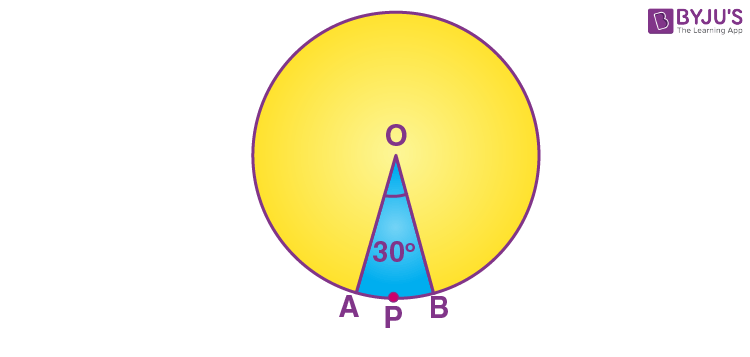Area of the major sector = [(360 – θ)/ 360] × πr2

=[(360 – 30)/360] × 3.14 × 4 × 4

= (330/360) × 3.14 × 16

= 46.05 cm2

= 46.1 cm2 (approx)

Q.4: Find the area of the segment AYB shown in the figure, if the radius of the circle is 21 cm and ∠ AOB = 120°. (Use π = 22/7).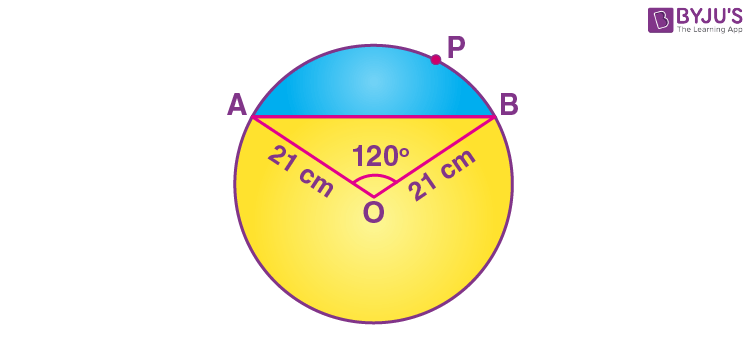Solution:

Area of the segment AYB = Area of sector OAYB – Area of ∆ OAB …..(1)

Area of the sector OAYB = (120/360) × (22/7) × 21 × 21 = 462 cm2 ……(2)

Draw OM ⊥ AB.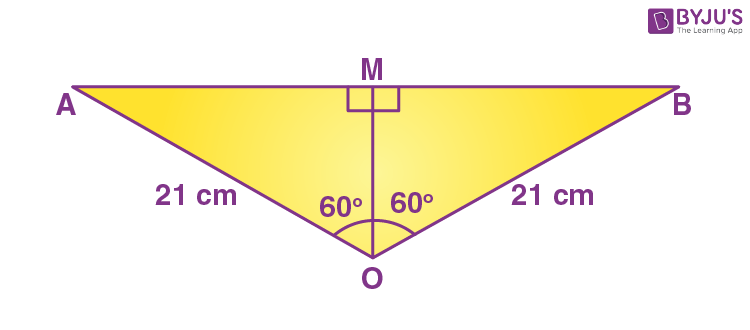Therefore, by RHS congruence, ∆ AMO ≅ ∆ BMO.

M is the mid-point of AB and ∠ AOM = ∠ BOM = (1/2) × 120° = 60°

Let OM = x cm

In triangle OMA,

OM/OA = cos 60°

x/21 = ½

x = 21/2

OM = 21/2 cm

Similarly,

AM/OA = sin 60°

AM/21 = √3/2

AM = 21√3/2 cm

AB = 2 AM = 2 (21√3/2) = 21√3 cm

Area of triangle OAB = (½) × AB × OM

= (½) × 21√3 × (21/2)

= (441/4)√3 cm2 ………..(3)

From (1), (2) and (3),

Area of the segment AYB = [462 – (441/4)√3] cm2

Q.5: Find the area of the shaded design in the given figure, where ABCD is a square of 10 cm and semicircles are drawn with each side of the square as diameter. (Use π = 3.14).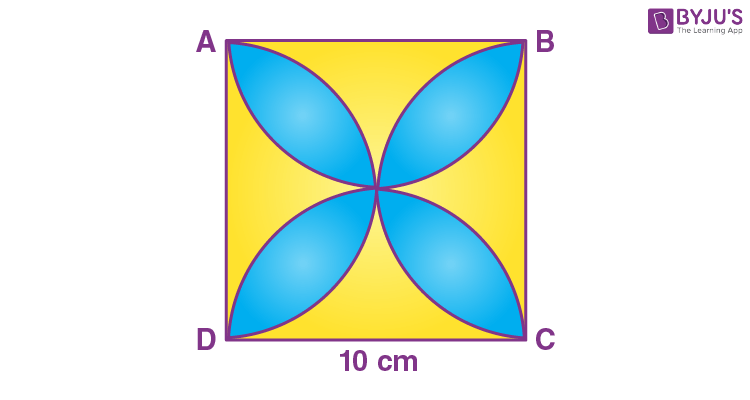Solution:

Let us assign I, II, III and IV for the unshaded regions.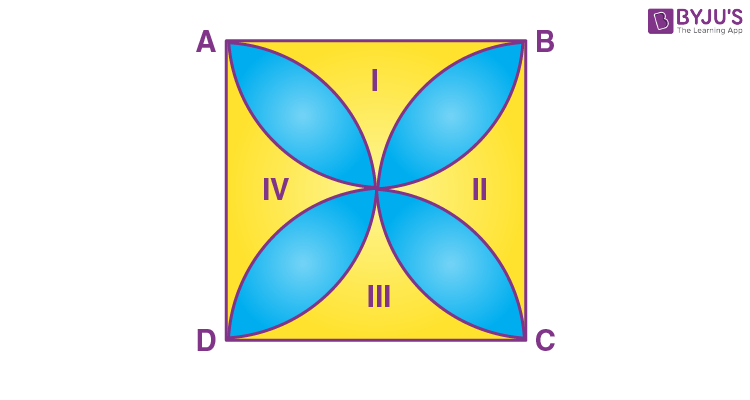Give that, side of square ABCD = 10 cm

The sides of a square are also the diameters of semicircles.

The radius of semicircle = 10/2 = 5 cm

Now, area of the region I +III = Area of square ABCD – Area of two semicircles of radius 5 cm

= (10)2 – 2 × (½) π ×(5)2

= 100 – 3.14 × 25

= 100 – 78.5

= 21.5 cm2

Similarly,

Area of the region II + Iv = 21.5 cm2

Area of the shaded region = Area of square ABCD – Area of the region (I + II + III + IV)

= 100 – 2× 21.5

= 100 – 43

= 57 cm2

Q.6: A round table cover has six equal designs as shown in the figure. If the radius of the cover is 28 cm, find the cost of making the designs at the rate of Rs. 0.35 per cm2. (Use 3 = 1.7)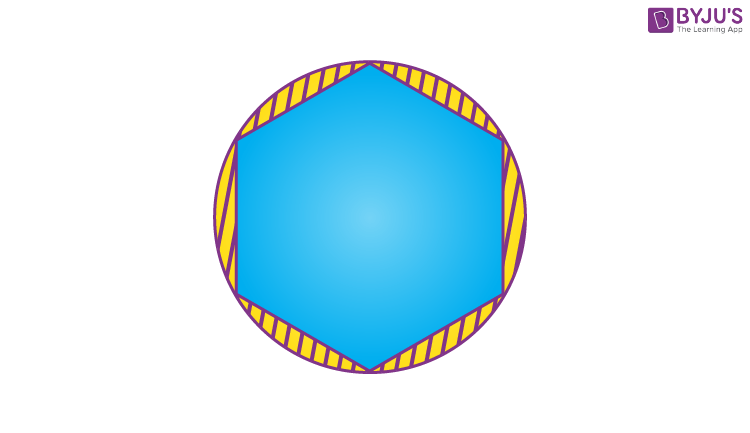Solution: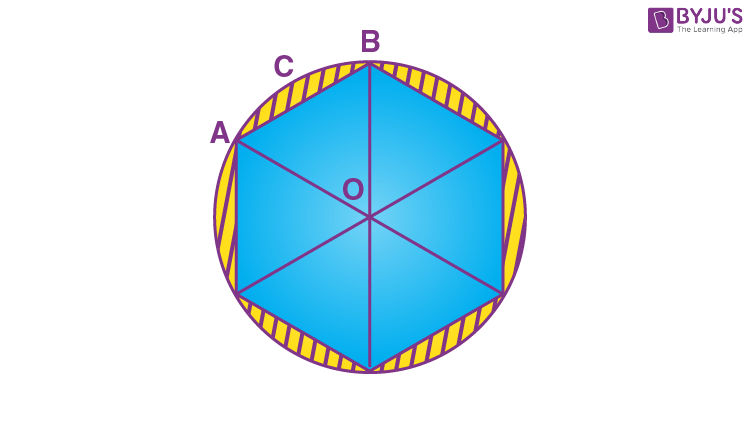Total number of equal designs = 6

∠AOB = 360°/6 = 60°

The radius of the cover = 28 cm

Cost of making design = Rs. 0.35 per cm2

Since the two arms of the triangle are the radii of the circle and thus are equal, and one angle is 60°, ΔAOB is an equilateral triangle. So, its area will be √3/4 × a2

Here, a = OA

∴ Area of equilateral ΔAOB = √3/4 × 282 = 333.2 cm2

Area of sector ACB = (60°/360°) × π r2 cm2

= 410.66 cm2

Area of a single design = area of sector ACB – Area of ΔAOB

= 410.66 cm2 – 333.2 cm2 = 77.46 cm2

∴ Area of 6 designs = 6 × 77.46 cm2 = 464.76 cm2

So, the total cost of making design = 464.76 cm2 × Rs. 0.35 per cm2

= Rs. 162.66

Q.7: In the figure, AB and CD are two diameters of a circle (with centre O) perpendicular to each other and OD is the diameter of the smaller circle. If OA = 7 cm, find the area of the shaded region (pink and yellow regions together).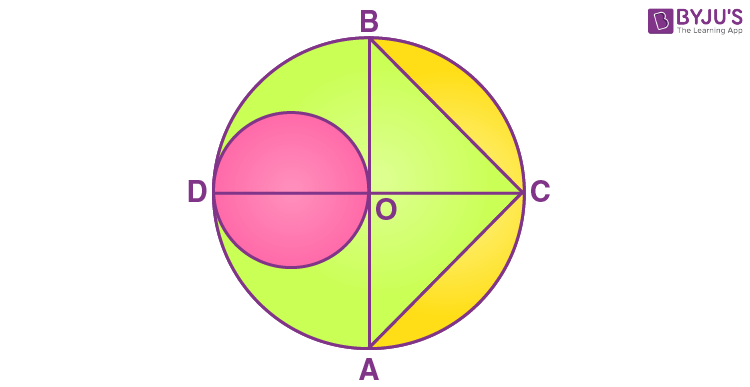Solution:

Radius of larger circle, R = 7 cm

Radius of smaller circle, r = 7/2 cm

Height of ΔBCA = OC = 7 cm

Base of ΔBCA = AB = 14 cm

Area of ΔBCA = 1/2 × AB × OC = 1/2 × 7 × 14 = 49 cm2

Area of larger circle = πR2 = 22/7 × 72 = 154 cm2

Area of larger semicircle = 154/2 cm2 = 77 cm2

Area of smaller circle = πr2 = 22/7 × 7/2 × 7/2 = 77/2 cm2

Area of the shaded region = Area of the larger circle – Area of a triangle – Area of larger semicircle + Area of the smaller circle

Area of the shaded region = (154 – 49 – 77 + 77/2) cm2

= 66.5 cm2

Q.8:  In the figure, O is the centre of a circle such that diameter AB = 13 cm and AC = 12 cm. BC is joined. Find the area of the shaded region. (take π = 3.14)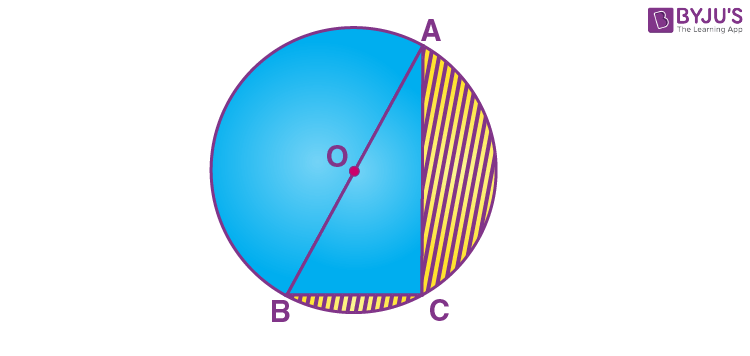Solution:

We know that the angle in the semicircle is the right angle.

Thus, ∠ACB = 9°

By Pythagoras theorem,

BC2 + AC2 = AB2

BC2 = AB2 – AC2

= (13)2 – (12)2

= 169 – 144

= 25

⇒ BC = 5 cm

From the given,

Diameter of circle = AB = 13 cm

Radius of semicircle = AB/2 = 13/2 cm

Area of the shaded region = Area of the semicircle – Area of right triangle ABC

= (1/2)πr2 – (1/2) × BC × AC

= (1/2) × 3.14 × (13/2) × (13/2) – (1/2) × 5 × 12

= 66.33 – 30

= 36.33 cm2

### Practice Questions For Class 10 Maths Chapter 12 Areas Related to Circles

1. A calf is tied with a rope of length 6 m at the corner of a square grassy lawn of side 20 m. If the length of the rope is increased by 5.5m, find the increase in the area of the grassy lawn in which the calf can graze.
2. Find the radius of a circle whose circumference is equal to the sum of the circumferences of two circles of radii 15 cm and 18 cm.
3. In the given figure, ABC is a quadrant of a circle of radius 14 cm and a semicircle is drawn with BC as diameter. Find the area of the shaded (in yellow colour) region.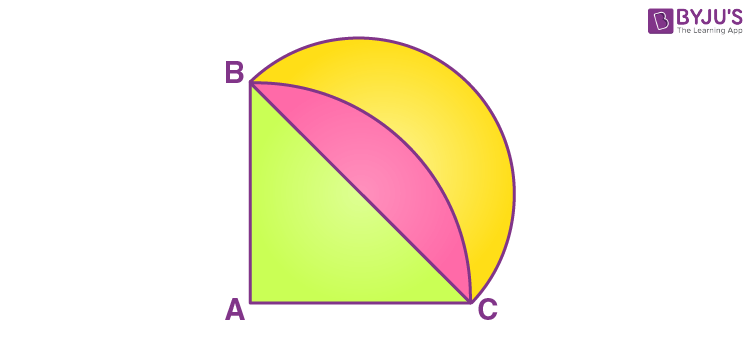4. Find the area of the minor segment of a circle of radius 14 cm, when the angle of the corresponding sector is 60°.
5. If the difference between the circumference and the radius of a circle is 37 cm, then using π = 22/7, calculate the circumference (in cm) of the circle.
6. The length of the minute hand of a clock is 14 cm. Find the area swept by the minute hand in 5 minutes.
7. A pipe of wire 22 cm long is bent into the form of an arc of a circle subtending an angle of 60° at its centre. Find the radius of the circle. [use π = 22/7]
8. Find the area of the shaded region in the figure, where arcs are drawn with centres A, B, C and D intersect in pairs at midpoints P, Q, R and S of the sides AB, BC, CD and DA respectively of a square ABCD of side 12 cm. [use π = 3.14]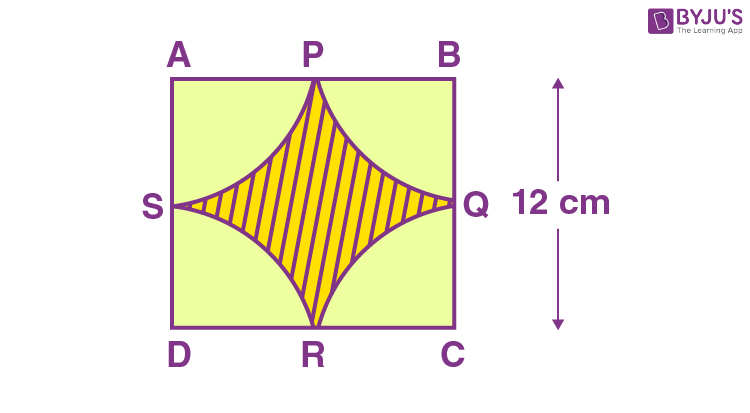9. Three semicircles each of diameter 3 cm, a circle of diameter 4.5 cm and a semicircle of radius 4.5 cm are drawn in the given figure. Find the area of the shaded region.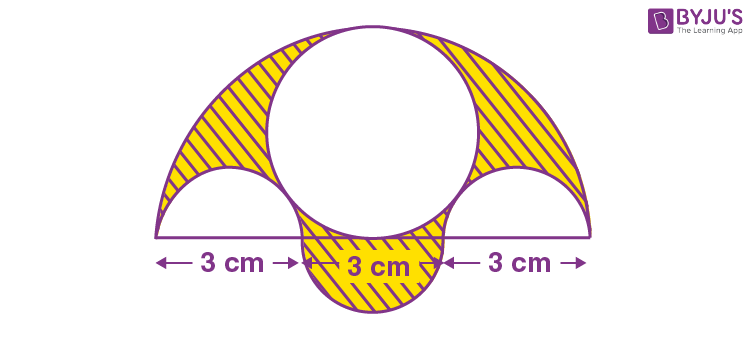10. In the figure, a square OABC is inscribed in a quadrant OPBQ. If OA = 15 cm, find the area of the shaded region. (use π = 3.14)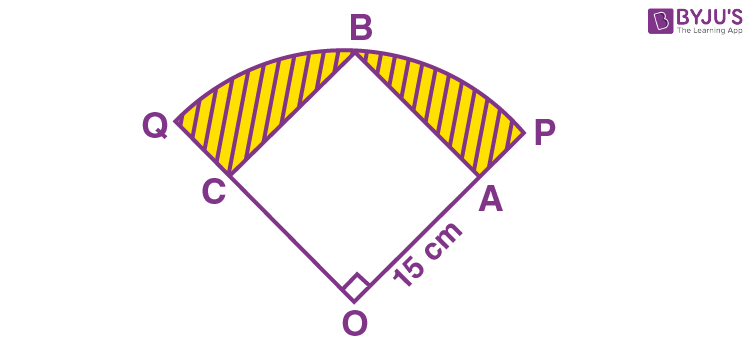## Video Lessons on Circles

### Introduction to Circles### Parts of a Circle### Area of a Circle